# Team:XMU Software/Project/terminator

PROJECT
Our project includes 2 independent software tools-the brick worker and E' NOTE. The former is a software suit for the evaluation and optimization of biobricks, i.e., promoter, RBS, protein coding sequences and terminator. E' NOTE is a web application serving as an assistant for experiments. Its useful functions such as experiments recording and experimental template customization make experimental process easier and more enjoyable.Terminator

# Background

Gene expression in both prokaryotes and eukaryotes is frequently controlled at the level of transcription. This process can be represented as a cycle consisting of four major steps: (1) promoter binding; (2) RNA chain initiation; (3) RNA chain elongation; and (4) termination. Since regulatory controls are exerted at each step, an understanding of the mechanism of each step is of general importance in understanding gene expression.

In the promoter part of our program, we've discussed the mechanism of promoter binding step and how it affects the transcription level. To complete our biobrick evaluation program and to better understanding of transcription process, we integrated the software developed by 2012 iGEM team SUSTC-Shenzhen-B to realize the prediction of transcription termination efficiency.

# Introduction

Termination, the last step of the transcription cycle, occurs when the RNA polymerase releases the RNA transcript and dissociates from the DNA template. It is important that transcription is imperfectly terminated at some terminator so that the ratio of the amount of the mRNA transcribed from upstream and that from downstream of the terminator is controlled. This regulation is quantified by the termination efficiency (%T).

Two mechanisms of transcription termination and two classes of termination signals have been described in bacteria: rho-dependent and rho-independent.

Rho-independent (also known as intrinsic) terminators are sequences motifs found in many prokaryotes that cause the transcription of DNA to RNA to stop. These termination signals typically consist of a short, often GC-rich hairpin followed by a sequences enriched in thymine residues.

The conventional model of transcriptional termination is that the stem loop causes RNA polymerase to pause and transcription of the poly-A tail causes the RNA: DNA duplex to unwind and dissociate from RNA polymerase.

# Algorithm

In 2011, iGEM team SUSTC-Shenzhen-B developed a software tool TTEC to predict terminator efficiency. It takes DNA sequences as input and returns the terminator efficiency value.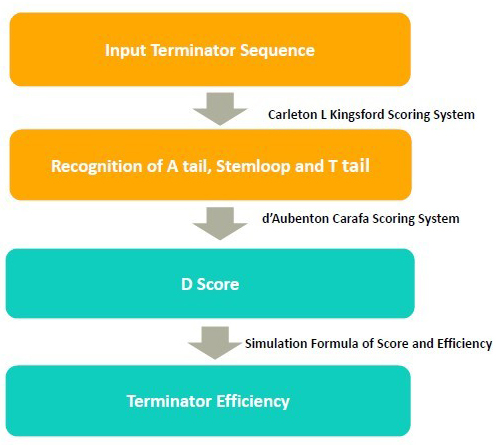In the algorithm, it takes 3 steps to calculate the terminator efficiency:

1. Use RNA folding algorithm to predict the secondary structure of terminator and and recognize A tail, stemloop and T tail.

2. From the secondary structure, we calculate the free energy of stem loop, and generate a score by considering stem loop free energy and T tail

3. From the score, we predict the terminator efficiency based on the score-terminator equation.

The prediction of secondary and recognition of A tail, stemloop and T tail are achieved by Kingsford scoring system.

# Kingsford Scoring System

In 2007, Carleton L. Kingsford et al. described TransTermHP1, a new computational method to rapidly and accurately detect Rho-independent transcription terminators.

They put forward an algorithm to predict Rho-independent terminators. The first 15 bases of the potential tail sequences are scored using a function: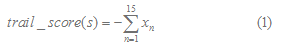where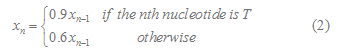for n=1,2,..,15 and =1.

The energy of potential hairpin configurations adjacent to a reference position can be found efficiently with a dynamic programming algorithm. The table entry hairpin_score[i,j] gives the cost of the best hairpin structure for which the base of the 5' stem is at nucleotide position i and the base of the 3' stem is at position j. The entry hairpin_score[i,j] can be computed recursively as follows: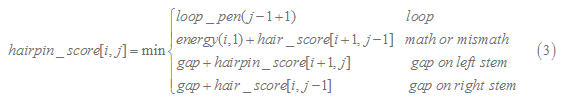The function energy(i,j) gives the cost of pairing the nucleotide at i with that at j, and loop_pen(n) gives the cost of a hairpin loop of length n. The hairpin's loop is forced to have a length between 3 and 13 nt, inclusive, by setting loop_pen(n) to a large constant for any n outside that range. The constant 'gap' gives the cost of not pairing a base with some base on the opposite stem and thus introducing a gap on one side of the hairpin stem.

Table 1

Parameters used to evaluate hairpins

Pairing Energy

G-C -2.3

A-T -0.9

G-T 1.3

Mismatch 3.5

Gap 6.0

Loop_pen(n) 1•(n - 2)

Parameters used to evaluate the energy of a potential hairpin where n is the length of the hairpin loop

The D score is calculated by Carafa Scoring System.

# Carafa Scoring System

Scoring System 2 is based on the model created by d'Aubenton Carafa 2. The score of terminator consists of two parts, the free energy of stemloop and the score of 15 nt poly T tail. The free energy of stemloop is calculated using Loop Dependent Energy Rules 3. The minimization of the free energy also determined the secondary structure of the stemloop. T tail score is calculated by the formula given by d' Aubenton Carafa.

Detailed Calculation of Score

### 1. Some definitions3

i. Closing Base Pair

For an RNA sequences, we number it from 5' to 3' . If i < j and nucleotides ri and rj form a base pair,we denote it by i.j. We call base ri' or base pair i'.j' is accessible from i.j if i <i' ( <j' ) <j and if there is no other base pair k.l so that i <k <i' ( <j' ) <l <j. We denote the collection of base and base pair accessible from i.j by L(i,j). Then i.j is the closing base pair. Here “L” means loop.

ii. n-loop

If the loop contain n – 1 base pairs, we denote it by n-loop. (Because there is a closing base pair, so we denote it by n-loop even though the closing base pair is not included in the loop.)

Here we can divide loops which may be formed in the terminator secondary structure into two kinds.

1-loop : Hairpin loop(size of loop shouldn't be smaller than 3)

2-loop : Interior Loop(right strand size and left strand size are both bigger than 0.)

Buldge(Size of one strand is bigger than 0 and that of another strand is 0.)Stack(size of the loop is 0.)

### 2. Calculation of the Minimum Free Energy Change of Stemloop Formation4 Assume i.j is the closing base pair of the loop

G（i,j）= min { GH ( i , j ) , GS( i , j ) + G ( i + 1 , j – 1 ) , GBI( i , j ) } ;

GBI ( i , j ) = min{ gbi( i , j , k , l ) + G( k , l ) } for all 0 < k – i + l – j - 2 < max_size

G(i,j) is the minimum free energy change of stemloop formation. GH is the free energy change to form a hairpin loop. GS is the free energy change to form a stack. GBI is to calculate the minimum free energy change of structure containing 2-loop. gbi(i,j,k,l) is the free energy change to form 2-loop.

### 3.Calculation of T Tail Score

Here we consider 15 nucleotide in the downstream of stemloop. T tail score nT is calculated as follows :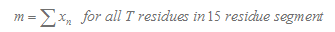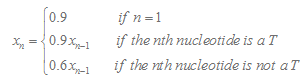In our program, if the length of the T tail( n ) is less than 15, we will only consider n nucleotides. If TL is more than 15, we will only consider 15 nucleotides.

### 4.Calculation of Score

Score = nT * 18.16 + deltaG / LH * 96.59 – 116.87

Here nT is T tail score. deltaG is the minimum free energy change of stemloop formation. LH is the length of stemloop.5,6

# References

 Kingsford, C. L.; Ayanbule, K.; Salzberg, S. L., Rapid, accurate, computational discovery of Rho-independent transcription terminators illuminates their relationship to DNA uptake. Genome biology 2007, 8 (2), R22.
 Carafa, Y. d. A.; Brody, E.; Thermes, C., Prediction of rho-independent E. coli transcription terminators: A statistical analysis of their RNA stem-loop structures. Journal of molecular biology 1990,216 (4), 835-858.
 Manual of Mfold Version 3.5.
 http://unafold.math.rpi.edu/lectures/old_RNAfold/node2.html.
 Lesnik, E. A.; Sampath, R.; Levene, H. B.; Henderson, T. J.; McNeil, J. A.; Ecker, D. J., Prediction of rho-independent transcriptional terminators in E. coli.Nucleic acids research 3583-3594.
 Sugimoto, N.; Nakano, S.-i.; Katoh, M.; Matsumura, A.; Nakamuta, H.; Ohmichi, T.; Yoneyama, M.; Sasaki, M., Thermodynamic parameters to predict stability of RNA/DNA hybrid duplexes. Biochemistry 1995, 34 (35),11211-11216.